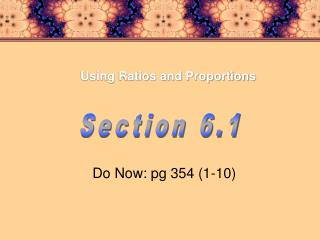Download PresentationUsing Ratios and Proportions

Using Ratios and Proportions - PowerPoint PPT Presentation

Using Ratios and Proportions . Section 6.1. Do Now: pg 354 (1-10). Vocabulary. Using Ratios and Proportions . What You'll Learn. You will learn to use ratios and proportions to solve problems. 1) ratio 2) proportion 3) cross products 4) extremes 5) MeansI am the owner, or an agent authorized to act on behalf of the owner, of the copyrighted work described.
Download PresentationUsing Ratios and Proportions

Download Policy: Content on the Website is provided to you AS IS for your information and personal use and may not be sold / licensed / shared on other websites without getting consent from its author.While downloading, if for some reason you are not able to download a presentation, the publisher may have deleted the file from their server.

- - - - - - - - - - - - - - - - - - - - - - - - - - E N D - - - - - - - - - - - - - - - - - - - - - - - - - -
Presentation Transcript
1. Using Ratios and Proportions Section 6.1 Do Now: pg 354 (1-10)

2. Vocabulary Using Ratios and Proportions What You'll Learn You will learn to use ratios and proportions to solve problems. • 1) ratio • 2) proportion • 3) cross products • 4) extremes • 5) Means • 6) Geometric Mean

3. Using Ratios and Proportions In 2000, about 180 million tons of solid waste was created in the United States. The paper made up about 72 million tons of this waste. The ratio of paper waste to total waste is 72 to 180. This ratio can be written in the following ways. 72 to 180 72:180 72 ÷ 180 a to b a:b a ÷ b where b  0

4. Using Ratios and Proportions Simplify the Ratio If the units of measure are the same then you reduce the ratio. If the units of measure are different you have to make them the SAME then reduce. 64m : 6 m Because both units are the same all you do is reduce the fraction. 32 :3

5. Using Ratios and Proportions 5 ft / 20 in Because the units are different I must FIRST make the units the same then reduce.

6. Using Ratios and Proportions proportion A __________ is an equation that shows two equivalent ratios. Every proportion has two cross products. In the proportion to the right, the terms 20 and 3 are called the extremes, and the terms 30 and 2 are called the means. = 20(3) 30(2) The cross products are 20(3) and 30(2). 60 = 60 equal The cross products are always _____ in a proportion.

7. Using Ratios and Proportions Likewise,

8. Using Ratios and Proportions Solve each proportion: = (30 – x)2 = 30(6) 3(x) 15(2x) 3x = 60 – 2x 30x = 180 5x = 60 x = 6 x = 12

9. Using Ratios and Proportions 6 16/5 12

10. Driving gear Driven gear equivalent ratio givenratio = driving gear driving gear = driven gear driven gear x = 14 Using Ratios and Proportions The gear ratio is the number of teeth on the driving gear to the number of teeth on the driven gear. If the gear ratio is 5:2 and the driving gear has 35 teeth, how many teeth does the driven gear have? 35 5 x 2 5x = 70 The driven gear has 14 teeth.

11. Ratios and Proportions

12. Using Ratios and Proportions 18\/3 18 12\/2

13. Using Ratios and Proportions End of Section 6.1 Homework: pg 360 3-51 odd